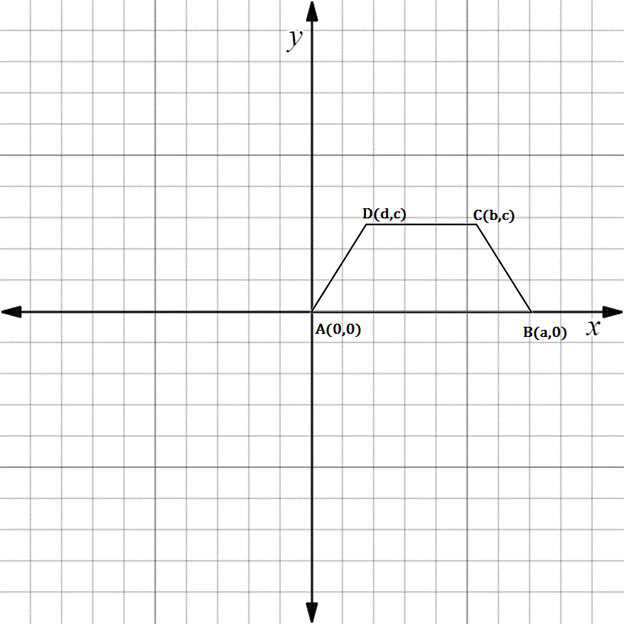Chapter 10.3, Problem 8EElementary Geometry For College St...

7th Edition
Alexander + 2 others
ISBN: 9781337614085

Solutions

Chapter
SectionElementary Geometry For College St...

7th Edition
Alexander + 2 others
ISBN: 9781337614085
Textbook Problem

In Exercises 5 to 10, the real numbers a, b, c, and d are positive.Consider the quadrilateral with vertices at A 0 ,   0 , B a ,   0 , C b ,   c , and D d ,   c . Explain why A B C D is a trapezoid.To determine

To explain:

ABCD is a trapezoid.

Explanation

The given diagram is shown below.

To prove ABCD is a trapezoid, we have to prove the following statement.

Only one pair of opposite sides are parallel.

AB is parallel to DC.

That is slope of AB is equal to slope of DC.

AD is not parallel to BC.

That is slope of AD is not equal to slope of BC.

Find the slope of AB.

x1,y1=A0, 0

x2,y2=Ba, 0

Formula for slope joining the line segment.

Slope is denoted by m.

m=y2-y1x2-x1

Here x1x2

Substituting the x and y co-ordinates.

mAB=0-0a-0

mAB=0

Then, find the slope of DC.

x1,y1=Dd, c

x2,y2=Cb, c

Substituting the x and y co-ordinates.

mDC=c-cb-d

mDC=0b-d

mDC=0

mAB=mDC

Hence, AD and BC are parallel

Still sussing out bartleby?

Check out a sample textbook solution.

See a sample solution

The Solution to Your Study Problems

Bartleby provides explanations to thousands of textbook problems written by our experts, many with advanced degrees!

Get Started

Define the terms population and sample, and explain the role of each in a research study.

Essentials of Statistics for The Behavioral Sciences (MindTap Course List)

For j – 2k 3i – 4k 3i + j – 2k 0 (zero vector)

Study Guide for Stewart's Multivariable Calculus, 8th

What is a ceiling effect, and how can it be a problem?

Research Methods for the Behavioral Sciences (MindTap Course List)

True or False: converges.

Study Guide for Stewart's Single Variable Calculus: Early Transcendentals, 8th